Learn data science and machine learning by building real-world projects on Jovian

## Exploring SVHN with CNN

SVHN(Street View House Number) Dataset is a real world dataset which is obtained from the Google Street View Images. This Dataset contains over 600,000 digit images. This Dataset is most likely big brother of MNIST Dataset which is "hello world " dataset for deep Learning.

This dataset full of some harder to recognise images of digits than MNIST. This dataset have two type of data, first full images and another cropped images.

We are going to explore this dataset and build an deep Neural Network using CNN(Convolution Neural Network).

### Dataset Overview

according to source ,

SVHN from stanford

http://ufldl.stanford.edu/housenumbers/

1. 10 classes, 1 for each digit. Digit '1' has label 1, '9' has label 9 and '0' has label 10.

2. 73257 digits for training, 26032 digits for testing, and 531131 additional, somewhat less difficult samples, to use as extra training data

**This dataset also available in Pytorch Torchvision datasets. In this project we use from torchvision dataset **

https://pytorch.org/docs/stable/torchvision/datasets.html

Before going to start let's install jovian so we can save our work

This project have following steps :

• importing required libraries
• exploring images of dataset
• Understanding convolution and pooling layers
• Creating a convolutional neural network (CNN) using PyTorch
• Training a CNN from scratch and monitoring performance
• Underfitting, overfitting and how to overcome them

### Importing necessary libraries

In :
``````import torch
import torchvision
import numpy as np
import matplotlib.pyplot as plt
import torch.nn as nn
import torch.nn.functional as F
from torchvision.datasets import SVHN
from torchvision.transforms import ToTensor
from torchvision.utils import make_grid
from torch.utils.data import random_split
%matplotlib inline
``````

let's give a name to our project which will be title to jovian notebook

In :
``project_name='svhn-cnn'``

Since our dataset contains images. but for a neural network we need input data in form of tensor.so we need to convert images dataset into pytorch tensor by using torchvision.transform module.

let's import it.

In :
``from torchvision.transforms import ToTensor``

generally dataset have spitted into two part.

1. train dataset
2. test dataset

In :
`` dataset = SVHN(root='data/', download=True, transform=ToTensor())``
```Using downloaded and verified file: data/train_32x32.mat ```

Let's look at a sample element from the training dataset. Each element is a tuple, containing a image tensor and a label. Since the data consists of 32x32 px color images with 3 channels (RGB), each image tensor has the shape `(3, 32, 32)`.

In :
``````img, label = dataset
print(img.shape, label)

``````
```torch.Size([3, 32, 32]) 1 ```

We can view the image using `matplotlib`, but we need to change the tensor dimensions to `(32,32,3)`. Let's create a helper function to display an image and its label.

In :
``````import matplotlib
import matplotlib.pyplot as plt
%matplotlib inline

matplotlib.rcParams['figure.facecolor'] = '#ffffff'``````
In :
``````def show_example(img, label):
print('Label: ', label)
plt.imshow(img.permute(1, 2, 0))``````

Let's look at a couple of images from the dataset. As you can tell, the 32x32px images are quite difficult to identify, even for the human eye. Try changing the indices below to view different images.

In :
``show_example(*dataset)``
```Label: 1 ```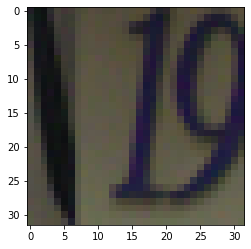In :
``show_example(*dataset)``
```Label: 4 ```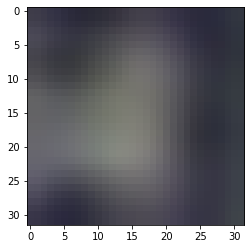In :
``show_example(*dataset)``
```Label: 7 ```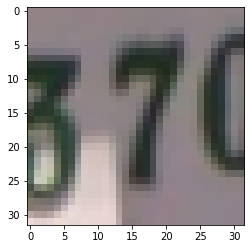Jovian offers an easy way of saving and sharing your Jupyter notebooks online.

so we are going to upload our notebook using jovian commit

In :
``!pip install jovian --upgrade -q``
In :
``import jovian``
In :
``jovian.commit(project=project_name)``
```[jovian] Detected Colab notebook... [jovian] Uploading colab notebook to Jovian... [jovian] Capturing environment.. [jovian] Attaching records (metrics, hyperparameters, dataset etc.) [jovian] Committed successfully! https://jovian.ai/proprincekush/svhn-cnn ```
Out:
``'https://jovian.ai/proprincekush/svhn-cnn'``

`jovian.commit` uploads the notebook to your Jovian account, captures the Python environment, and creates a shareable link for your notebook, as shown above. You can use this link to share your work and let anyone (including you) run your notebooks and reproduce your work.

### Training and Validation Datasets

While building real world machine learning models, it is quite common to split the dataset into 3 parts:

1. Training set - used to train the model i.e. compute the loss and adjust the weights of the model using gradient descent.
2. Validation set - used to evaluate the model while training, adjust hyperparameters (learning rate etc.) and pick the best version of the model.
3. Test set - used to compare different models, or different types of modeling approaches, and report the final accuracy of the model.

Since there's no predefined validation set, we can set aside a small portion (5000 images) of the training set to be used as the validation set. We'll use the `random_split` helper method from PyTorch to do this. To ensure that we always create the same validation set, we'll also set a seed for the random number generator.

In :
``````random_seed = 42
torch.manual_seed(random_seed);
``````
In :
``````val_size = 5000
train_size = len(dataset) - val_size

train_ds, val_ds = random_split(dataset, [train_size, val_size])
len(train_ds), len(val_ds)``````
Out:
``(68257, 5000)``

Let's record `dataset_url`, `val_pct` and `rand_seed` using `jovian.log_dataset`.

In :
``jovian.log_dataset(dataset_url='http://ufldl.stanford.edu/housenumbers/train_32x32.mat', val_size=val_size, random_seed=random_seed)``
```[jovian] Dataset logged. ```

We can now create data loaders for training and validation, to load the data in batches

In :
``````from torch.utils.data.dataloader import DataLoader

batch_size=128``````

we are going to work with a training dataset,so we insure that it is properly randomize . we set `shuffle=true`. we also use the power of gpu and their number of core so we enabled `pin-memory` and set `num_workers` values

for the validation dataset we can use higher batch size due to no need to work eith gradient

In :
``````train_dl = DataLoader(train_ds, batch_size, shuffle=True, num_workers=4, pin_memory=True)
val_dl = DataLoader(val_ds, batch_size*2, num_workers=4, pin_memory=True)``````

We can look at batches of images from the dataset using the `make_grid` method from `torchvision`. Each time the following code is run, we get a different bach, since the sampler shuffles the indices before creating batches.

In :
``````
from torchvision.utils import make_grid

def show_batch(dl):
for images, labels in dl:
fig, ax = plt.subplots(figsize=(12, 6))
ax.set_xticks([]); ax.set_yticks([])
ax.imshow(make_grid(images, nrow=16).permute(1, 2, 0))
break``````
In :
``show_batch(train_dl)``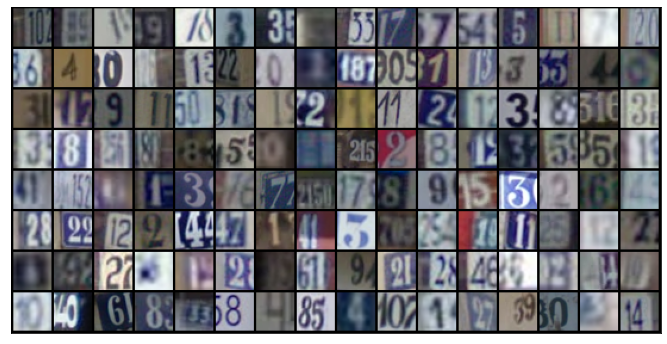Once again, let's save and commit our work using `jovian` before proceeding further.

In :
``````import jovian
jovian.commit(project=project_name, environment=None)``````
```[jovian] Detected Colab notebook... [jovian] Uploading colab notebook to Jovian... ```
```--------------------------------------------------------------------------- ApiError Traceback (most recent call last) <ipython-input-42-403a3b2e6848> in <module>() 1 import jovian ----> 2 jovian.commit(project=project_name, environment=None) /usr/local/lib/python3.6/dist-packages/jovian/utils/commit.py in commit(message, files, outputs, environment, privacy, filename, project, new_project, git_commit, git_message, **kwargs) 134 return 135 --> 136 res = perform_colab_commit(project, privacy) 137 slug, username, version, title = res['slug'], res['owner']['username'], res['version'], res['title'] 138 /usr/local/lib/python3.6/dist-packages/jovian/utils/colab.py in perform_colab_commit(project, privacy) 45 if res.status_code == 200: 46 return res.json()['data'] ---> 47 raise ApiError('Colab commit failed: ' + pretty(res)) ApiError: Colab commit failed: (HTTP 500) The server encountered an internal error and was unable to complete your request. Either the server is overloaded or there is an error in the application.```

### Defining the Model (Convolutional Neural Network)

we will use a convolutional neural network, using the `nn.Conv2d` class from PyTorch.

The 2D convolution is a fairly simple operation at heart: you start with a kernel, which is simply a small matrix of weights. This kernel “slides” over the 2D input data, performing an elementwise multiplication with the part of the input it is currently on, and then summing up the results into a single output pixel. - Source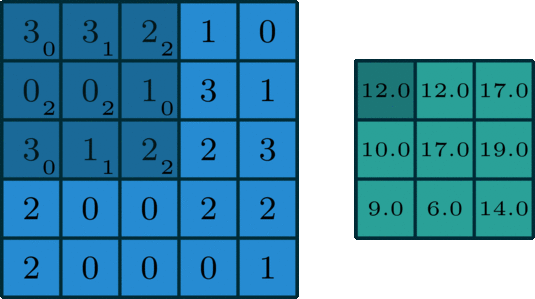Let us implement a convolution operation on a 1 channel image with a 3x3 kernel.

In :
``````def apply_kernel(image, kernel):
ri, ci = image.shape       # image dimensions
rk, ck = kernel.shape      # kernel dimensions
ro, co = ri-rk+1, ci-ck+1  # output dimensions
output = torch.zeros([ro, co])
for i in range(ro):
for j in range(co):
output[i,j] = torch.sum(image[i:i+rk,j:j+ck] * kernel)
return output``````
In :
``````sample_image = torch.tensor([
[3, 3, 2, 1, 0],
[0, 0, 1, 3, 1],
[3, 1, 2, 2, 3],
[2, 0, 0, 2, 2],
[2, 0, 0, 0, 1]
], dtype=torch.float32)

sample_kernel = torch.tensor([
[0, 1, 2],
[2, 2, 0],
[0, 1, 2]
], dtype=torch.float32)

apply_kernel(sample_image, sample_kernel)
``````
Out:
``````tensor([[12., 12., 17.],
[10., 17., 19.],
[ 9.,  6., 14.]])``````

For multi-channel images, a different kernel is applied to each channels, and the outputs are added together pixel-wise.

There are certain advantages offered by convolutional layers when working with image data:

• Fewer parameters: A small set of parameters (the kernel) is used to calculate outputs of the entire image, so the model has much fewer parameters compared to a fully connected layer.
• Sparsity of connections: In each layer, each output element only depends on a small number of input elements, which makes the forward and backward passes more efficient.
• Parameter sharing and spatial invariance: The features learned by a kernel in one part of the image can be used to detect similar pattern in a different part of another image.

We will also use a max-pooling layers to progressively decrease the height & width of the output tensors from each convolutional layer.The `Conv2d` layer transforms a 3-channel image to a 16-channel feature map, and the `MaxPool2d` layer halves the height and width. The feature map gets smaller as we add more layers, until we are finally left with a small feature map, which can be flattened into a vector. We can then add some fully connected layers at the end to get vector of size 10 for each image.Let's define the model by extending an `ImageClassificationBase` class which contains helper methods for training & validation.

In :
``````class ImageClassificationBase(nn.Module):
def training_step(self, batch):
images, labels = batch
out = self(images)                  # Generate predictions
loss = F.cross_entropy(out, labels) # Calculate loss
return loss

def validation_step(self, batch):
images, labels = batch
out = self(images)                    # Generate predictions
loss = F.cross_entropy(out, labels)   # Calculate loss
acc = accuracy(out, labels)           # Calculate accuracy
return {'val_loss': loss.detach(), 'val_acc': acc}

def validation_epoch_end(self, outputs):
batch_losses = [x['val_loss'] for x in outputs]
epoch_loss = torch.stack(batch_losses).mean()   # Combine losses
batch_accs = [x['val_acc'] for x in outputs]
epoch_acc = torch.stack(batch_accs).mean()      # Combine accuracies
return {'val_loss': epoch_loss.item(), 'val_acc': epoch_acc.item()}

def epoch_end(self, epoch, result):
print("Epoch [{}], train_loss: {:.4f}, val_loss: {:.4f}, val_acc: {:.4f}".format(
epoch, result['train_loss'], result['val_loss'], result['val_acc']))

def accuracy(outputs, labels):
_, preds = torch.max(outputs, dim=1)

We'll use `nn.Sequential` to chain the layers and activations functions into a single network architecture.

In :
``````class SVHNCnnModel(ImageClassificationBase):
def __init__(self):
super().__init__()
self.network = nn.Sequential(
nn.ReLU(),
nn.ReLU(),
nn.MaxPool2d(2, 2), # output: 64 x 16 x 16

nn.ReLU(),
nn.ReLU(),
nn.MaxPool2d(2, 2), # output: 128 x 8 x 8

nn.ReLU(),
nn.ReLU(),
nn.MaxPool2d(2, 2), # output: 256 x 4 x 4

nn.Flatten(),
nn.Linear(256*4*4, 1024),
nn.ReLU(),
nn.Linear(1024, 512),
nn.ReLU(),
nn.Linear(512, 10))

def forward(self, xb):
return self.network(xb)``````
In :
``````model = SVHNCnnModel()
model
``````
Out:
``````SVHNCnnModel(
(network): Sequential(
(0): Conv2d(3, 32, kernel_size=(3, 3), stride=(1, 1), padding=(1, 1))
(1): ReLU()
(2): Conv2d(32, 64, kernel_size=(3, 3), stride=(1, 1), padding=(1, 1))
(3): ReLU()
(4): MaxPool2d(kernel_size=2, stride=2, padding=0, dilation=1, ceil_mode=False)
(5): Conv2d(64, 128, kernel_size=(3, 3), stride=(1, 1), padding=(1, 1))
(6): ReLU()
(7): Conv2d(128, 128, kernel_size=(3, 3), stride=(1, 1), padding=(1, 1))
(8): ReLU()
(9): MaxPool2d(kernel_size=2, stride=2, padding=0, dilation=1, ceil_mode=False)
(10): Conv2d(128, 256, kernel_size=(3, 3), stride=(1, 1), padding=(1, 1))
(11): ReLU()
(12): Conv2d(256, 256, kernel_size=(3, 3), stride=(1, 1), padding=(1, 1))
(13): ReLU()
(14): MaxPool2d(kernel_size=2, stride=2, padding=0, dilation=1, ceil_mode=False)
(15): Flatten(start_dim=1, end_dim=-1)
(16): Linear(in_features=4096, out_features=1024, bias=True)
(17): ReLU()
(18): Linear(in_features=1024, out_features=512, bias=True)
(19): ReLU()
(20): Linear(in_features=512, out_features=10, bias=True)
)
)``````

Let's verify that the model produces the expected output on a batch of training data. The 10 outputs for each image can be interpreted as probabilities for the 10 target classes (after applying softmax), and the class with the highest probability is chosen as the label predicted by the model for the input image.

In :
``````for images, labels in train_dl:
print('images.shape:', images.shape)
out = model(images)
print('out.shape:', out.shape)
print('out:', out)
break``````
```images.shape: torch.Size([128, 3, 32, 32]) out.shape: torch.Size([128, 10]) out: tensor([-0.0298, 0.0386, 0.0380, 0.0193, 0.0271, 0.0093, 0.0356, 0.0401, -0.0428, -0.0168], grad_fn=<SelectBackward>) ```

To seamlessly use a GPU, if one is available, we define a couple of helper functions (`get_default_device` & `to_device`) and a helper class `DeviceDataLoader` to move our model & data to the GPU as required.

In :
``````def get_default_device():
"""Pick GPU if available, else CPU"""
if torch.cuda.is_available():
else:

def to_device(data, device):
"""Move tensor(s) to chosen device"""
if isinstance(data, (list,tuple)):
return [to_device(x, device) for x in data]
return data.to(device, non_blocking=True)

"""Wrap a dataloader to move data to a device"""
def __init__(self, dl, device):
self.dl = dl
self.device = device

def __iter__(self):
"""Yield a batch of data after moving it to device"""
for b in self.dl:
yield to_device(b, self.device)

def __len__(self):
"""Number of batches"""
return len(self.dl)``````

Based on where you're running this notebook, your default device could be a CPU (`torch.device('cpu')`) or a GPU (`torch.device('cuda')`)

In :
``````device = get_default_device()
device
``````
Out:
``device(type='cuda')``

We can now wrap our training and validation data loaders using `DeviceDataLoader` for automatically transferring batches of data to the GPU (if available), and use `to_device` to move our model to the GPU (if available).

In :
``````train_dl = DeviceDataLoader(train_dl, device)
to_device(model, device);
``````

Once again, let's save and commit the notebook before we proceed further.

In :
``jovian.commit(project=project_name)``
```[jovian] Detected Colab notebook... [jovian] Uploading colab notebook to Jovian... [jovian] Capturing environment.. [jovian] Attaching records (metrics, hyperparameters, dataset etc.) [jovian] Committed successfully! https://jovian.ai/proprincekush/svhn-cnn ```
Out:
``'https://jovian.ai/proprincekush/svhn-cnn'``

### Training the Model

We'll define two functions: `fit` and `evaluate` to train the model using gradient descent and evaluate its performance on the validation set. For a detailed walkthrough of these functions.

In :
``````@torch.no_grad()
model.eval()
outputs = [model.validation_step(batch) for batch in val_loader]
return model.validation_epoch_end(outputs)

history = []
optimizer = opt_func(model.parameters(), lr)
for epoch in range(epochs):
# Training Phase
model.train()
train_losses = []
loss = model.training_step(batch)
train_losses.append(loss)
loss.backward()
optimizer.step()
# Validation phase
result['train_loss'] = torch.stack(train_losses).mean().item()
model.epoch_end(epoch, result)
history.append(result)
return history``````

Before we begin training, let's instantiate the model once again and see how it performs on the validation set with the initial set of parameters.

In :
``model = to_device(SVHNCnnModel(), device)``
In :
``evaluate(model, val_dl)``
Out:
``{'val_acc': 0.0902918204665184, 'val_loss': 2.302222967147827}``

The initial accuracy is vary around 7%-14%, which is what one might expect from a randomly intialized model (since it has a 1 in 10 chance of getting a label right by guessing randomly).

We'll use the following hyperparmeters (learning rate, no. of epochs, batch_size etc.) to train our model.

In :
``````num_epochs = 10
lr = 0.001``````

It's important to record the hyperparameters of every experiment you do, to replicate it later and compare it against other experiments. We can record them using `jovian.log_hyperparams`.

In :
``````jovian.reset()
jovian.log_hyperparams({
'num_epochs': num_epochs,
'opt_func': opt_func.__name__,
'batch_size': batch_size,
'lr': lr,
})
``````
```[jovian] Hyperparams logged. ```
In :
``history = fit(num_epochs, lr, model, train_dl, val_dl, opt_func)``
```Epoch , train_loss: 2.0105, val_loss: 0.9258, val_acc: 0.7010 Epoch , train_loss: 0.4966, val_loss: 0.3632, val_acc: 0.8937 Epoch , train_loss: 0.3118, val_loss: 0.3120, val_acc: 0.9053 Epoch , train_loss: 0.2513, val_loss: 0.2748, val_acc: 0.9144 Epoch , train_loss: 0.2110, val_loss: 0.2488, val_acc: 0.9259 Epoch , train_loss: 0.1755, val_loss: 0.2389, val_acc: 0.9288 Epoch , train_loss: 0.1541, val_loss: 0.2447, val_acc: 0.9320 Epoch , train_loss: 0.1243, val_loss: 0.2499, val_acc: 0.9328 Epoch , train_loss: 0.1089, val_loss: 0.2831, val_acc: 0.9209 Epoch , train_loss: 0.0921, val_loss: 0.3154, val_acc: 0.9207 ```
In [ ]:
``# history = fit(5, 1e-3, model, train_dl, val_dl, opt_func)``

Just as we have recorded the hyperparameters, we can also record the final metrics achieved by the model using `jovian.log_metrics` for reference, analysis and comparison.

In :
``````jovian.log_metrics(train_loss=history[-1]['train_loss'],
val_loss=history[-1]['val_loss'],
val_acc=history[-1]['val_acc'])``````
```[jovian] Metrics logged. ```

We can also plot the valdation set accuracies to study how the model improves over time.

In :
``````def plot_accuracies(history):
accuracies = [x['val_acc'] for x in history]
plt.plot(accuracies, '-x')
plt.xlabel('epoch')
plt.ylabel('accuracy')
plt.title('Accuracy vs. No. of epochs');``````
In :
``plot_accuracies(history)``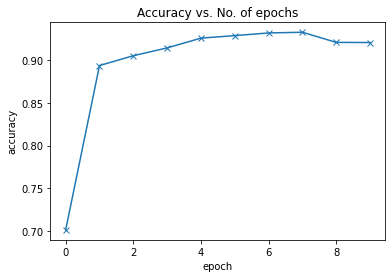Our model reaches an accuracy of around 92%, and by looking at the grap. This suggests that we might need to use a more powerful model to capture the relationship between the images and the labels more accurately. This can be done by adding more convolutional layers to our model, or incrasing the no. of channels in each convolutional layer, or by using regularization techniques.

We can also plot the training and validation losses to study the trend.

In :
``````def plot_losses(history):
train_losses = [x.get('train_loss') for x in history]
val_losses = [x['val_loss'] for x in history]
plt.plot(train_losses, '-bx')
plt.plot(val_losses, '-rx')
plt.xlabel('epoch')
plt.ylabel('loss')
plt.legend(['Training', 'Validation'])
plt.title('Loss vs. No. of epochs');``````
In :
``plot_losses(history)``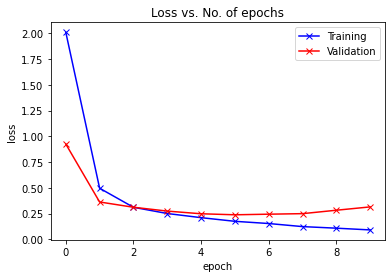Initialy, both the training and validation losses seem to decrease over time. However, if you train the model for long enough, you will notice that the training loss continues to decrease, while the validation loss stops decreasing, and even starts to increase after a certain point!This phenomenon is called overfitting, and it is the no. 1 why many machine learning models give rather terrible results on real-world data. It happens because the model, in an attempt to minimize the loss, starts to learn patters are are unique to the training data, sometimes even memorizing specific training examples. Because of this, the model does not generalize well to previously unseen data.

Following are some common stragegies for avoiding overfitting:

• Gathering and generating more training data, or adding noise to it
• Using regularization techniques like batch normalization & dropout
• Early stopping of model's training, when validation loss starts to increase

Before continuing, let us save our work to the cloud using `jovian.commit`.

In :
``jovian.commit(project=project_name)``
```[jovian] Detected Colab notebook... [jovian] Uploading colab notebook to Jovian... [jovian] Capturing environment.. [jovian] Attaching records (metrics, hyperparameters, dataset etc.) [jovian] Committed successfully! https://jovian.ai/proprincekush/svhn-cnn ```
Out:
``'https://jovian.ai/proprincekush/svhn-cnn'``

### Testing with individual images

While we have been tracking the overall accuracy of a model so far, it's also a good idea to look at model's results on some sample images. Let's test out our model with some images from the predefined test dataset of 10000 images. We begin by creating a test dataset using the `ImageFolder` class.

In :
``test_dataset = SVHN(root='data/', download=True,split='test' ,transform=ToTensor())``
```Downloading http://ufldl.stanford.edu/housenumbers/test_32x32.mat to data/test_32x32.mat ```
``HBox(children=(FloatProgress(value=1.0, bar_style='info', max=1.0), HTML(value='')))``

Let's define a helper function `predict_image`, which returns the predicted label for a single image tensor.

In :
``````def predict_image(img, model):
# Convert to a batch of 1
xb = to_device(img.unsqueeze(0), device)
# Get predictions from model
yb = model(xb)
# Pick index with highest probability
_, preds  = torch.max(yb, dim=1)
# Retrieve the class label
return preds``````
In :
``````img, label = test_dataset
plt.imshow(img.permute(1, 2, 0))
print('Label:', label, ', Predicted:', predict_image(img, model))``````
```Label: 5 , Predicted: tensor(, device='cuda:0') ```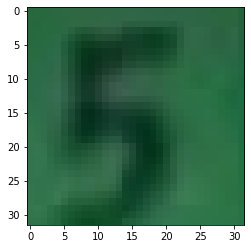In :
``````
img, label = test_dataset
plt.imshow(img.permute(1, 2, 0))
print('Label:', label, ', Predicted:', predict_image(img, model))``````
```Label: 9 , Predicted: tensor(, device='cuda:0') ```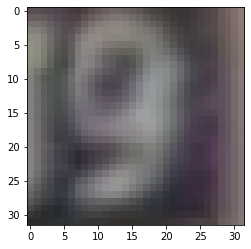In :
``````img, label = test_dataset
plt.imshow(img.permute(1, 2, 0))
print('Label:', label, ', Predicted:', predict_image(img, model))``````
```Label: 6 , Predicted: tensor(, device='cuda:0') ```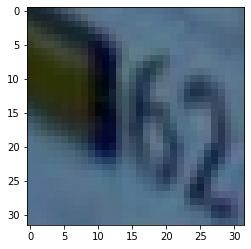Identifying where our model performs poorly can help us improve the model, by collecting more training data, increasing/decreasing the complexity of the model, and changing the hypeparameters.

As a final step, let's also look at the overall loss and accuracy of the model on the test set, and record using `jovian`. We expect these values to be similar to those for the validation set. If not, we might need a better validation set that has similar data and distribution as the test set (which often comes from real world data).

In :
``````test_loader = DeviceDataLoader(DataLoader(test_dataset, batch_size*2), device)
result
``````
Out:
``{'val_acc': 0.9140799045562744, 'val_loss': 0.3504485487937927}``
In :
``jovian.log_metrics(test_loss=result['val_loss'], test_acc=result['val_acc'])``
```[jovian] Metrics logged. ```

Since we've trained our model for a long time and achieved a resonable accuracy, it would be a good idea to save the weights of the model to disk, so that we can reuse the model later and avoid retraining from scratch. Here's how you can save the model.

In :
``torch.save(model.state_dict(), 'svhn-cnn.pth')``

The `.state_dict` method returns an `OrderedDict` containing all the weights and bias matrices mapped to the right attributes of the model. To load the model weights, we can redefine the model with the same structure, and use the `.load_state_dict` method.

Let's make one final commit using `jovian`.

In :
``jovian.commit(project=project_name,environment=None)``
```[jovian] Detected Colab notebook... [jovian] Uploading colab notebook to Jovian... [jovian] Attaching records (metrics, hyperparameters, dataset etc.) [jovian] Committed successfully! https://jovian.ai/proprincekush/svhn-cnn ```
Out:
``'https://jovian.ai/proprincekush/svhn-cnn'``
In [ ]:
`` ``